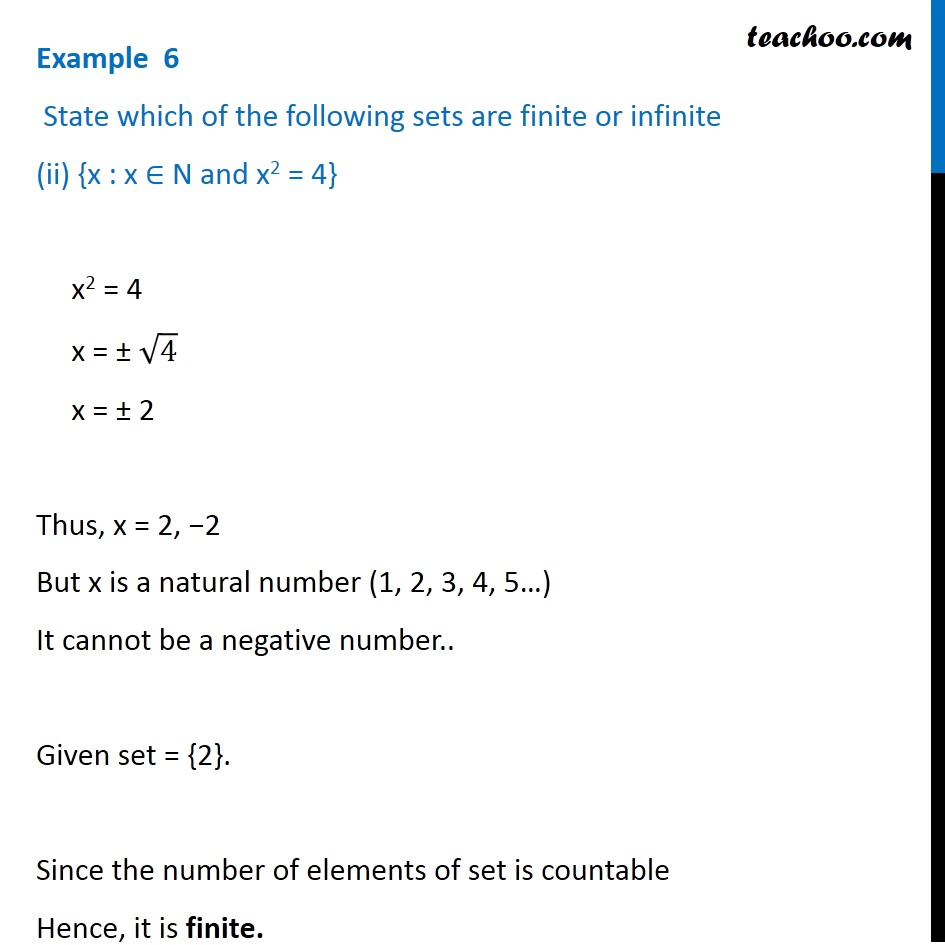Examples

Chapter 1 Class 11 Sets
Serial order wiseLearn in your speed, with individual attention - Teachoo Maths 1-on-1 Class

### Transcript

Example 6 State which of the following sets are finite or infinite (ii) {x : x ∈ N and x2 = 4} x2 = 4 x = ± √4 x = ± 2 Thus, x = 2, −2 But x is a natural number (1, 2, 3, 4, 5…) It cannot be a negative number.. Given set = {2}. Since the number of elements of set is countable Hence, it is finite.